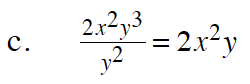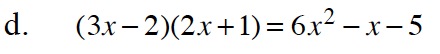### Home > CC4 > Chapter 8 > Lesson 8.1.6 > Problem8-89

8-89.

Determine which of the following equations are true for all values (always true). For those that are not, decide whether they are true for certain values (sometimes true) or not true for any values (never true). Justify your decisions clearly. Homework Help ✎

 a. (x − 5)2 = x2 + 25 b. (2x − 1)(x + 4) = 2x2 + 7x − 4 c.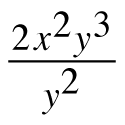= 2x2y d. (3x − 2)(2x + 1) = 6x2 − x − 5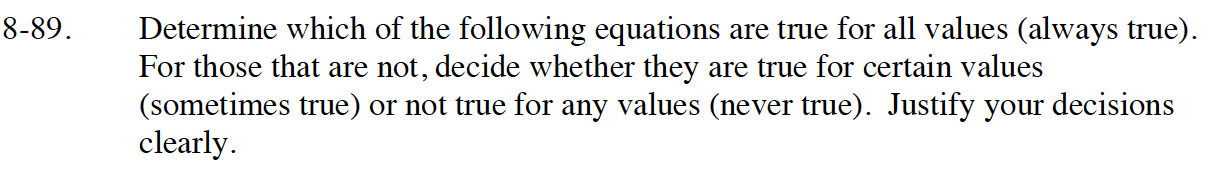Read Math Notes box for Lesson A.1.9 on number of solutions for a single variable equation. The principles still apply to this problem.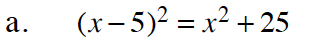Use a generic rectangle to multiply (x − 5)² = (x − 5)(x − 5)

Sometimes true. (when x = 0)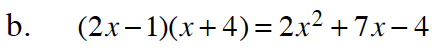Use a generic rectangle to multiply (2x − 1)(x + 4).